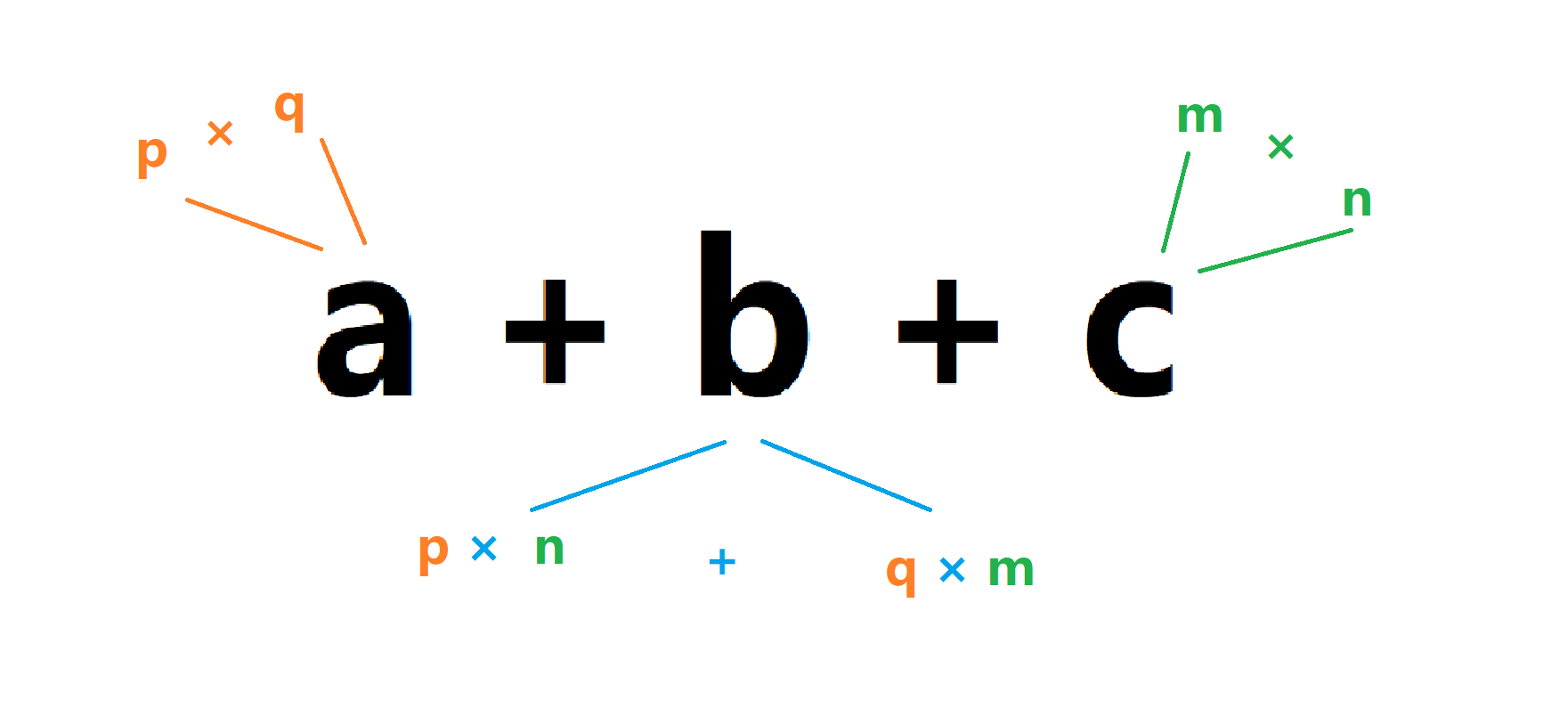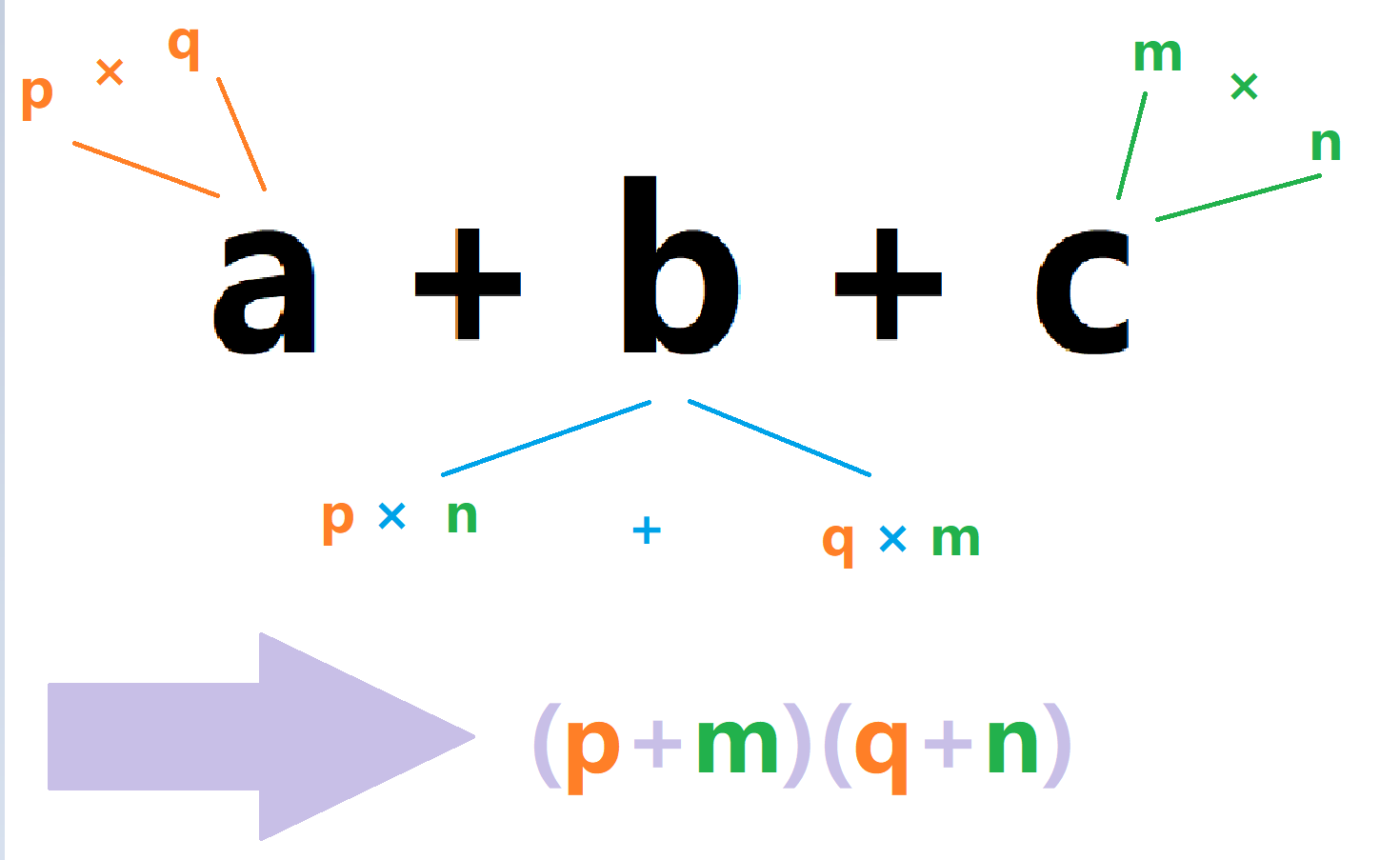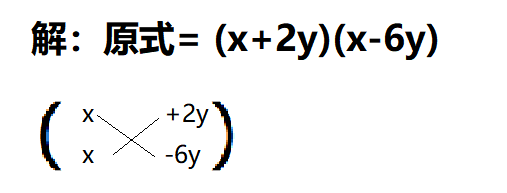# Part -1：幂的运算

$a^b\cdot a^c = a^{b+c}$
$\frac{a^b}{a^c} = a^{b-c}$
$(a^m)^n = a^{mn}$
$a^c\cdot b^c=(ab)^c$
$a^0 = 1(a \neq 0)$
$a^{-p}=\frac{1}{a^p}(a\neq 0)$

# Part 0：什么是因式分解

$c(a+b) = ac + bc$

# Part 1：因式分解第一招——乘法分配律！

$(1) 2a + 2b$
$(2) 2a^2 + 4ab$
$(3) 2ab + 2bc + 2abc$
$(4) 2ca + 2bc^2$

$\text{解：}(1): 2a+2b = 2(a+b)$

$2a^2+4ab = 2a\cdot a+ 2ab \cdot 2$

# Part 2：公式的运用

$a^2-b^2=(a+b)(a-b)$
$a^2+2ab+b^2=(a+b)^2$
$a^2-2ab+b^2=(a-b)^2$

$(1)(x-y)^2-4$

$a^2-b^2(a = x-y,b = 2)$

$(x-y+2)(x-y-2)$

$(x^2 - y^2)^2$

$(x^2 - y^2)^2$

$x^2-y^2$

$[(x-y)(x+y)]^2$

$(x-y)^2(x+y)^2$

# Part 3：项太多了怎么办？分组！

$xy+x+y+1$

$(x+1)y + (x+1)$

$(x+1)(y+1)$

# Part 4：特殊二次三项式的杀手：十字相乘# Part End：来自DBXXX大佬的友情提示：